# The Geometric Viewpoint

geometric and topological excursions by and for undergraduates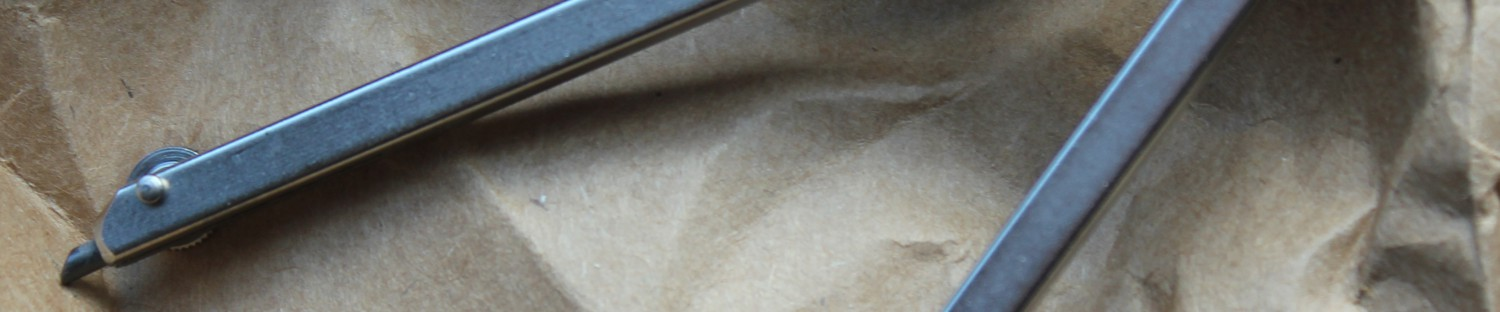• Tag Archives Hyperbolic Geometry
• ## History of Hyperbolic Geometry

Sami was a student in the Fall 2016 course “Geometry of Surfaces” taught by Scott Taylor at Colby College. The essay has been lightly edited before being published here.

Introduction
This essay is an introduction to the history of hyperbolic geometry. Euclid, Gauss, Felix Klein and Henri Poincare all made major contribution to the field. We will discuss some of their influences in the following sections, starting with Euclid and his postulates that defined geometry. Then we will look at the effect of Gauss’s thoughts on Euclid’s parallel postulate through noneuclidean geometry. Later, Klein settled any doubt of noneuclidean consistency. Lastly, Poincare makes some notable contributions to solidifying hyperbolic geometry as an area of academic study.

Euclid’s Postulates
Euclidean geometry came from Euclid’s five postulates. It is the most intuitive geometry in that it is the way humans naturally think about the world. Nonetheless, there are a few other lesser-known, but equally important, geometries that also have many applications in the world and the universe. These “other” geometries come from Euclid’s fifth postulate: “If a straight line falling on two straight lines makes the interior angles on the same side less than two right angles the two straight lines if produced indefinitely meet on that side on which the angles [are] less than two right angles” . See Figure 1(A) below for an illustration of this. An equivalent way to express this is that the angle sum of a triangle is two right angles.

This is also called the parallel postulate because it is equivalent to the following statement: “if one draws a straight line and a point not on that line, there is only one unique line that is parallel to given line through the point” . See Figure 1(B) below.

Unlike the other postulates, the fifth is not obvious (to satisfy your curiosity, the rest of Euclid’s postulates are attached at the end of this essay). For centuries, mathematicians and amateurs alike attempted to prove that the fifth postulate is a consequence of the first four postulates and other established theorems . However, it turns out this postulate determines whether we are in euclidean or noneuclidean geometry .

The Birth of Hyperbolic Geometry
Over 2,000 years after Euclid, three mathematicians finally answered the question of the parallel postulate. Carl F. Gauss, Janos Bolyai, and N.I. Lobachevsky are considered the fathers of hyperbolic geometry. Gauss published little of his work on it because he, allegedly, feared disrespecting Euclid by disproving the parallel postulate. Yet, Gauss extended geometry to include what he coined “non-euclidean,” or the contradiction of the parallel postulate . Bolyai and Lobachevsky published their works on noneuclidean geometry independently but around the same time; however, their findings were not popularized until after 1862, when Gauss’s private letter to Schumacher about his “meditations” on hyperbolic geometry was published. With the work of these three mathematicians, the controversy of the parallel postulate was put to rest. It was definitive that without the parallel postulate, the remaining four postulates created a geometry that is equally consistent .

What is hyperbolic geometry?
Now that a brief history of the sources of hyperbolic geometry has been provided, we will define hyperbolic geometry. It has constant negative Gaussian curvature, which resembles a hyperboloid (See Figure 2). Note, that spherical geometry has constant positive curvature .

First, we will give a few different ways to visualize hyperbolic geometry, then we will explain how to measure distance in this space.

Visualization of Hyperbolic Geometry
A more natural way to think about hyperbolic geometry is through a crochet model as shown in Figure 3 below. This discovery by Daina Taimina in 1997 was a huge breakthrough for helping people understand hyperbolic geometry when she crocheted the hyperbolic plane. This was important because now people can touch and move the crocheted object to get a more intuitive understanding of what a straight line in hyperbolic space would look like.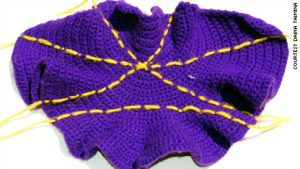Figure 3: A crochet from Daina Taimina . You can clearly see that the parallel postulate does not hold here because there are three lines that go through a point and none of them intersect with the given line on the bottom.

As mentioned before, we can visualize hyperbolic geometry through crochet. However, there are some different models including the upper-half plane and the Poincare disk model. See Figure 4 below. The upper-half plane model has the real line as the axis, which we may approach but will never reach. The disk model has the same idea as we approach the edge of the circle. Lines that appear to hit these ideal boundaries at the same point have an angle of 0 between them.

Definition of Distance
Although it may be a bit surprising at first, the definition of metric, or measure of distance, is different for each type of geometry. In Euclidean geometry, the distance is given as$d_{euc}= \sqrt{(x_1-x_0)^2+(y_1-y_0)^2}$

between two points$(x_0,y_0)$ and$(x_1,y_1)$. Note that in euclidean geometry, the shortest distance between two points is a line (as postulated by Euclid). However, in hyperbolic space, the shortest distance between two points is the arc from point$a$ to point$b$ that lie on the unique semicircle centered at a point on the boundary as shown in Figure 5; if we parameterize the arc as$t \mapsto (x(t),y(t)), \ \ a\leq t \leq b$,$l_{hyp} = \int_{a}^{b} \frac{\sqrt{x'(t)^2+y'(t)^2}}{y(t)} dt$

See .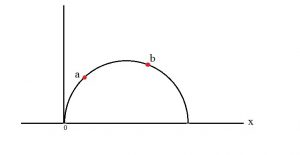Figure 5: Hyperbolic geodesic pictured as a euclidean semi-circle centered at a point on x.

Now that we’ve gone over the founding of hyperbolic geometry and have some sense of what it is, we’ll talk about two more influential mathematicians in the field: Felix Klein and Henri Poincare.

Felix Klein
Klein’s contribution to geometry is not only the famous Klein Bottle, but also his proof of the extension of the Cayley Measure on projective geometry to non-euclidean geometry. According to John Stillwell’s translation of On so-called Noneuclidean Geometry, Klein started by showing that each non-euclidean geometry is a special case of projective geometry; he then ended by concluding that non-euclidean geometry can be derived from projective geometry. Furthermore, projective geometry is independent from the theory of parallels; if not, the consistency of projective geometry would be questioned.

Projective Geometry

Projective geometry has simple origins from Renaissance artists who portrayed the rim of a cup as an ellipse in their paintings to show perspective. Projective geometry can be thought of in this way:

The theory of perspective regards the artist’s canvas as a transparent screen through which he looks from a fixed vantage point at the scene he is painting. Light rays coming from each point of the scene are imagined to enter his eye, and the totality of these lines is called a projection. 

From there, the idea eventually entered the academic mathematical community where it was incorporated into the study of linear algebra and other areas of mathematics. Projective geometry is the study of invariants on projections – properties of figures which are not modified in the process of projection . Projective geometry is more more general than both euclidean and hyperbolic geometries and this is what Klein uses to show that noneuclidean geometries are consistent.

Euclidean and hyperbolic geometry follows from projective geometry

Klein gives a general method of constructing length and angles in projective geometry, which he believed to be the fundamental concept of geometry. To borrow psychology terms, Klein’s approach is a top-down way to look at non-euclidean geometry while the upper-half plane, disk model and other models would be referred to as bottom-up methods. The bottom-up methods are easier to visualize and to deal with applications of hyperbolic geometry. This is remarkable because this top-down approach provides a “more comprehensive”  proof than those that had in a case by case manner. Moreover, projective geometry is consistent outside of geometry .

Distance in general
The different geometries we get from projective geometry come from the the projection of the fundamental conic. This idea is illustrated below in Figure 7.

First, Klein defines the general distance function as$2ic \text{ arccos }\frac{\Omega_{xy}}{\sqrt{\Omega_{xx} \Omega_{yy}}}$
where the constant$c$ determines which space we are in (Hyperbolic, parabolic, elliptic) and$\begin{array}{rcl}\Omega_{xx} &=& ax_1 ^2+bx_1x_2+cx_2 ^2\\ \Omega_{yy} &=& ay_1 ^2+by_1y_2+cy_2 ^2 \ \text{and} \\ \Omega_{xy} &=& ax_1y_1+b(x_1y_2+y_1x_2)+cx_2y_2 \end{array}$
with$(x_1,x_2) \text{ and } (y_1,y_2)$ as the coordinates of two points.

Klein shows that the fundamental conic section “corresponds completely with the idea of hyperbolic geometry.” Now, the fundamental conic is the projection onto the plane with the appropriate metric; it “determines a measure on all basic figures of the first kind in the plane”. Here, the “first kind” is fuchsian groups , which are isometries of the hyperbolic plane. An isometry is a way of transforming a figure in a given space without changing its angles or lengths. Every isometry in hyperbolic space can be written as a linear fractional transformation in the following form:$\phi(z) = \frac{az+b}{cz+d}$

where$z$ is the complex coordinate of a point. You can think of a complex coordinate in the same way that you think about$(x,y)$ on the euclidean plane where$z = x +\text{i}y$. The “basic figures” are the triangle, circle, and the square. So these isometries take triangles to triangles, circles to circles and squares to squares.

The fundamental conic that forms hyperbolic geometry is proper and real – but “we shall never reach the fundamental conic, let alone pass beyond it”. Recall, our visualizations of hyperbolic space using the upper-half plane model from Figure 4(A), then the fundamental conic is the real line and the fuchsian groups are the isometries acting on$\mathbb{H}^2$.

By using projective geometry to construct noneuclidean geometries, Klein has created a proof that is indisputable.

Henri Poincare
A discussion of the history of hyperbolic geometry cannot leave out Henri Poincare after which the Poincare disk model is named (See Figure 4 (B)). Originally, he began his studies in differential equations, then stumbled upon hyperbolic geometry naturally in his work. He wrote in his book Science and Methods that:

The incidents of travel made me forget my mathematical work. When we arrived at Countances, we got into a break to go for a drive, and, just as I put my foot on the step, the idea came to me, though nothing in my former thoughts seemed to have prepared me for it, that the transformations I had used to define Fuchsian functions were identical with those of non-euclidean geometry. 

In fact, he was studying the differential equation$x''(t)+p(t)x(t)=0$ for a given function$p(t)$ that resembles linear fractional transformations with real coefficients. When perturbed to have slightly complex coefficients, this yielded a group$\Gamma$ of linear fractional maps with complex coefficients that act on the Riemann Sphere. This is also related to chaos! He called the ones with real coefficients fuchsian groups after Fuchs and the ones with complex coefficients kleinian groups, though neither Fuchs nor Klein had much to do with the groups they are named after. Allegedly, Klein was offended when Poincare declared the fuchsian group, claiming it had been he, not Fuchs, who had thought about it. So to appease Klein, Poincare named the next group after him. Nonetheless, these are groups of isometries on the hyperbolic plane,$\mathbb{H}^2$ .

There have been many other theorems (and still more though current research) in hyperbolic space but these have been instrumental in the creation of hyperbolic geometry. Despite its turbulent history surrounding the parallel postulate and its late debut in the mathematical world, hyperbolic geometry is gaining prominence and has many great applications to the real world.

Euclid’s Postulates :

1. A straight line segment can be drawn joining any two points.
2. Any straight line segment can be extended indefinitely in a straight line.
3. Given any straight lines segment, a circle can be drawn having the segment as radius and one endpoint as center.
4. All Right Angles are congruent.
5. If two lines are drawn which intersect a third in such a way that the sum of the inner angles on one side is less than two Right Angles, then the two lines inevitably must intersect each other on that side if extended far enough.

References:

 Antonick, Gary. “The Non-Euclidean Geometry of Whales.” The New York Times. The New York Times, 08 Oct. 2012. Web. 04 Dec. 2016.
 Bonahon, Francis. Low-dimensional Geometry: From Euclidean Surfaces to Hyperbolic Knots. Providence, RI: American Mathematical Society, 2009. Print.
 Creates, Cris. “Crochet Mathematics: Hyperbolic Crochet.” Crochet Mathematics: Hyperbolic Crochet. N.p., 12 Jan. 2012. Web. 04 Dec. 2016.
 “Daina Taimina.” Daina Taimina | Crochet Coral Reef. N.p., n.d. Web. 04 Dec. 2016.
 Henderson, David W. Experiencing Geometry: In Euclidean, Spherical, and Hyperbolic Spaces. Contributer: Daina Taimina. 2nd ed. Upper Saddle River, NJ: Prentice Hall, 2001. Print.
 Lewis, Florence P. “History of the Parallel Postulate.” The American Mathematical Monthly, vol. 27, no. 1, 1920, pp. 16–23. www.jstor.org/stable/2973238.
 McMullen, Curtis T. “Euclid’s Postulates.” Euclid’s Postulates. Harvard University, 1997. Web. 07 Dec. 2016.
 Nicoguaro. Gaussian Curvature. Digital image. Wikicommons, 9 Jan. 2016. Web. 6 Dec. 2016.
 Poincaré, Henri. Science and Methods. Trans. Francis Maitland. London: T Nelson, 1914. Archive.org.
 Stillwell, John. “Introduction and Translation to Klein’s On the So-called Noneuclidean Geometry.” Sources of Hyperbolic Geometry. Vol. 10. Providence, RI: American Mathematical Society, 1996. 63-111. Print. History of Mathematics.
 Wylie, C. R. “Introduction to Projective Geometry.” Google Books. Courier Corporation, 2011. Web. 08 Dec. 2016.

This entry was posted in Hyperbolic Geometry and tagged , , . Bookmark the permalink.

• ## Completeness on an Incomplete Sphere.

I used to think I had a fairly good understanding of distance. If asked to find the distance between two points, I mentally connect them with a line segment and then find its length using the Pythagorean Theorem. Though I knew how to create fancy mathematical spaces, when it came to the real world I had my real sense of distance: straight lines connecting points.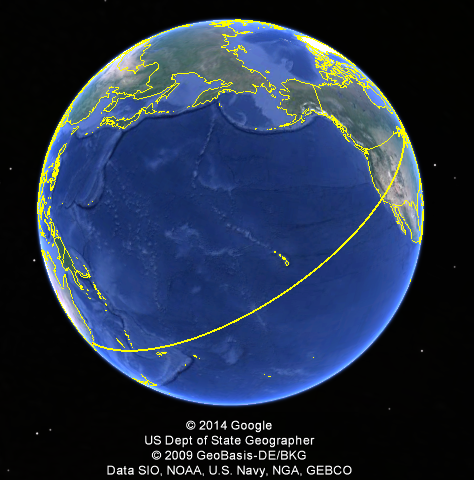Figure 1: When measuring the distance between cities, it doesn’t make sense to find the length along the straight line through the center of the Earth. Instead, we look at the length of the curve along the Earth’s surface.

Despite this intuition, I jettison this definition of distance when it doesn’t make sense. When I pulled out my ruler on Google Maps to find the distance between my hometown of Jay, Maine and Sydney, Australia, I found that there were over ten thousand miles between them. Quite impressive since the diameter of the Earth (the greatest distance between two points) is only about eight thousand miles. Clearly then, this “distance” according to Google is not just the linear displacement. In fact, as we see in Figure 1, this distance follows a path along the exterior of the sphere. Though different that our original idea of distance, this length is more useful if we were to plan a trip.

Suddenly, these fancy mathematical spaces with their unintuitive notions of distance didn’t seem quite so pointless. Depending on the purpose of the measurements, there is very good reason to draw something besides a straight line, or to even cause our sense of length to stretch as depending on our position. When working on my thesis, I encountered an interesting theorem by Paul Montel, which states that a set of analytic functions is normal when their range misses at least three points. In this proof, I was required to work with an entirely new sense of distance on this thrice punctured sphere.

In different contexts, we may define many different ways to measure distance (metrics) so that our measurements will be useful. One property we seek when defining a new metric is the idea of completeness. If we take a sequence of points,$( x_1, x_2,$…), completeness says that if the sum of the distances between each$x_n$ and the next one is finite, then the points must be getting arbitrarily close to some limit in our space. When traveling across the surface of the Earth, if we walk some finite distance and then stop we are always guaranteed to still be on the surface of the Earth. Completeness serves as an important property in real analysis. In fact, it is because we want completeness that we construct the real numbers from the rational numbers.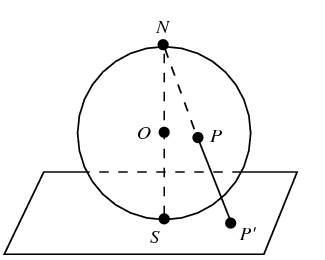Figure 2: The sphere is projected onto the plane by drawing a line from N through a point P, sending P to where the line crosses the plane (P’).

If we cut out some small, closed region from our sphere, or even a single point, then our new metric space (under the same definition of distance) ceases to be complete. Consider if a country, on Earth, closed itself to foreigners. I would never be able to travel to this country, despite the fact that the distance between me and its border would be finite. If we want to consider the Earth, without this country, to be a complete system, we must redefine distance so that the distance from anywhere on our space to the border of this country is infinite. To illustrate how we might define such a distance, let us look at a sphere which has a single point$N$ removed from it. We may transform any point of the once-punctured sphere onto the Euclidean ($\mathbb R^2$) plane via the continuous bijection known as the Stereographic Projection (Figure 2). We then say that the distance between two points on the sphere, p and q, is the Euclidean distance between p’ and q’. This metric, it turns out, is complete on the once- punctured sphere. Through a similar process, we may induce a metric on the a sphere with two distinct punctures using the Eucledian metric (we won’t go into detail about that process here).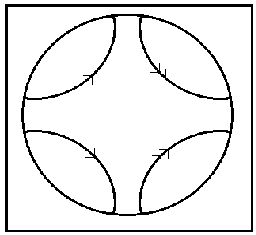Figure 3: Starting on the disk, we see that we can connect sides to make a pair of pants.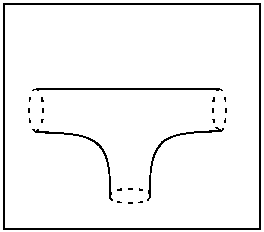Figure 4: The pair of pants can be deformed into a sphere with three holes.

So then, if we have a simple example of a metric on the sphere, and a way to induce a metric on the spheres with one or two punctures using Euclidean space, is it possible to find a complete  metric on the thrice-punctured sphere? The answer is yes… but not by relating our back to Euclidean space.

Consider the shape, S, formed by four arcs in a unit disk and its edge connecting them (Figure 3). Getting rid of the unnecessary portion of the disk, we may fold S over the x axis and connect the top two arcs to the bottom two. We then take any two points along these arcs sharing an x-coordinate and define them to be equivalent (”gluing” them together so to speak).  This gluing forms what we call a pair of pants. Note that this shape has three holes in it, suggesting a similarity to the thrice punctured sphere. In fact, we can stretch it so that it exactly resembles such a sphere (Figure 4). By making the arcs intersect the edge of the sphere closer to one another, we may shrink these punctures down to single points.

Recall how we defined a metric on the punctured sphere by sending points to the Euclidean plane. We now define distance on the thrice-punctured using the mapping from the sphere back into the disk. Note that, for any path on the sphere, we may unwrap the sphere back into$S$, giving us some collection of paths in the disk. We then define the length of a path by however long its segments are on the unwrapped space. The distance between two points, then, is the largest value which is shorter than the lengths of all these paths.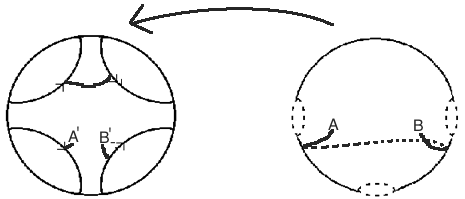Figure 5: Showing how a path on the thrice-punctured sphere can be “unwrapped” back onto our original shape S. The length of the path then, from A to B, is the length of each of the segments of its inverse image in S.

This just means that we need to clarify our notion of distance on$S$, inside of the open unit disk. Now, we could use the Euclidean metric again, but this would still fail to give us a complete space. If I take the sequence$0, \frac 12, \frac 23, \frac 34,\frac 45$…, it should not be hard to see that the sum of the distances between consecutive points is just one. Indeed, the first$n$ many pairs of distances will just be the difference between zero and the$n+1$th term. As the sequence goes to one, the sum of these lengths goes to one. However, the fact that the sequence converges to one also causes a problem, as one corresponds with one of the punctures on our sphere. So then, we cannot have a complete sense of distance on the open disk using the Euclidean sense of distance.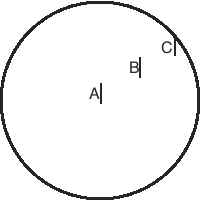Figure 6: Taking three line segments A,B, and C of equal Euclidean length, it turns out that the hyperbolic length of them is increased the closer they are to the boundary. The length of B is greater than that of A, while we say that the length of C is infinite, as it approaches the boundary.

This is where hyperbolic space comes in. On the unit disk model, we think of hyperbolic space as becoming squished near the boundary. Though the Euclidean distance between two points in the disk may only be some value d, if we slide them towards the edge of the disk (without changing their Euclidean displacement) then the hyperbolic distance between them approaches infinity. Though a severe over-simplification, think about taking a 6″ pen and holding it horizontally in front of your face. Though we might say that the pen has a constant Euclidean length, regardless of where you place it, if you slowly move the pen towards one of your eyes (while holding the other shut) the width of the pen appears to grow arbitrarily large, until it is wider than your field of vision.

It is this property that makes our space complete. Looking back at our previous example, of the sequence converging to one. In Euclidean space, the distances between consecutive terms added up to one. However, we now have that the magnitude of these distances gets amplified as they get closer to one. In fact, since the line segment from zero to one has infinite length in hyperbolic space, we infer that the sum of these distances (which are just the pieces of this line segment) must also grow arbitrarily large as we add them. We see then that this fixes the problem we had when we tried to apply a Euclidean manifold.

By repairing this issue, it turns out that our new space is, in fact, complete. The existence of a complete metric induced by the hyperbolic disk is implied by applying the Poincare’ Polygon Theorem to$S$. Some may wonder then, why am I making such a big deal about completeness? It turns out that many forms of advanced mathematics may only work to their full potential on complete spaces. My entire preoccupation with the thrice punctured sphere began when doing research for my honors thesis in Complex Analysis. Despite being a completely different field, I needed this definition of distance. The Schwarz–Ahlfors–Pick Theorem directly relates this metric to the idea of differentiability, allowing us to create differentiable functions on the open disk to this punctured sphere which don’t increase hyperbolic distance.

This entry was posted in Uncategorized and tagged , . Bookmark the permalink.

• ## Curvy Television

Television screen size became a status symbol in the American household soon after TV’s adoptionin the 1940’s, and remains so today. There are many equivalent ways to characterize screen size; today’s standard is by the diagonal length of the screen. Everyone understands that this length is related in a familiar way to a screen’s area. With today common 16:9 aspect ratio (horizontal length:vertical length), the screen area${A(l)=\alpha l^2}$ where${l}$ is the diagonal length and${\alpha=337/144}$. In other words, the area varies with the square of the diagonal length.

This polynomial relationship, however, is a feature of euclidean space. TV screens placed on hyperbolic and spherical surfaces lead to surprising differences in area relative to diagonal length. Curvature will play an important role in illustrating the discrepancy between euclidean, spherical, and hyperbolic geometries. To see this, let us imagine covering our television screen with a piece of paper. Using Ilmari Karonen’s imagery from Stack Exchange we shall lay a large sheet of paper over our euclidean television, and cut it down to size.

Now imagine that the surface of our TV is lying on a sphere. We will try to lay our cut-to-size sheet over the screen, a curved surface. To anyone who has used paper-mache, it’s immediately apparent that the paper will need to wrinkle and fold up around itself to fit to the surface. There is simply too much paper and too little sphere to go around. What is happening? The intuitive answer is that there is simply not enough space on our spherical surface. This simple fact hints at a sobering idea for cartographers; there exists no flat map that is locally isometric to the Earth at any point. No matter how carefully you restrict your vision, a flat map will be always be wrong. Gauss formally proves this in his Theorema Egregium. It turns out to be a consequence of the constant positive curvature of spherical space, as opposed to zero curvature in euclidean space.

Curvature, also know as Gaussian curvature${K}$ at a point${\alpha}$ on a surface, is defined as the product${\kappa_1 \cdot \kappa_2 }$, where${\kappa_1}$ and${\kappa_2 }$ are the principal curvatures of${\alpha}$. Finding the principal curvatures at${\alpha}$ can be visualized by the following method: First, find the normal vector to the surface at${\alpha}$. Next, look at the intersection of a plane containing the normal vector with the surface. This intersection is a curve on the surface. As you rotate the plane around the normal vector, the intersection curve will change. The principal curvatures are the maximum and minimum curvature of the intersection curve at${\alpha}$ as you rotate the plane.

On a plane, the intersection of a normal plane to a point on the surface will be a line. Since the curvature of any line is zero,${k_1 \cdot k_2 = 0}$ at all points, resulting in constant zero curvature as we have already mentioned. Similarly, on a sphere, any plane containing a normal vector will intersect the sphere along a great circle (geodesic) of the sphere. Since any two great circles have the same (non-zero) curvature, we see that${\kappa_1 \cdot \kappa_2 = \beta > 0}$ at all points, i.e. a sphere has constant positive Gaussian curvature.

Hyperbolic space challenges this definition of curvature. How can we use our method on a surface which cannot be embedded in euclidean 3-space? One way to visualize hyperbolic space is as a collection of points each of which locally looks like a saddle point. If we take a plane containing a normal vector to a saddle point, and intersect it with the saddle, we see that the curve on the saddle radically changes as we rotate the plane. The maximal and minimal curvatures of the intersection curves will be positive and negative respectively. Therefore,${K= \kappa_1 \cdot \kappa_2 < 0}$. Since each point of hyperbolic space locally looks like an identical saddle, we see that hyperbolic space has constant negative curvature.

What happens when we embed our television in a hyperbolic surface? The first thing to realize is that${2\pi}$ is the maximum area of a rectangular screen. This is similar to how a spherical rectangle is bounded by the size of the sphere. However, we do not want to restrict the size of our television. Bigger TV’s are always better! Instead, we will investigate the area of circular televisions, or hyperbolic disks, which have no upper bound. If we calculate the circumference of a hyperbolic circle in terms of its radius${r}$, we find that${Circ(r) = 2\pi\sinh(r)}$. Now we can simply integrate this value from${0}$ to${R}$ to find the area${A(R)}$ of a hyperbolic disk,${\int_0^R 2\pi\sinh(r) dr = 4\pi\sinh^2(\frac{1}{2}R)}$. This can be rewritten as${A(R) = \pi(e^{R}+e^{-R})-2\pi}$, where${R}$ is the radius of the disk.

Notice that in hyperbolic geometry, circular area grows exponentially with the radius of a disk. Our TV’s area, therefore, will grow much faster as we increase its radius than it would in euclidian space. In other words, there is more space in a hyperbolic disk than in a euclidean disk of the same radius. This is the opposite of our result from spherical geometry, and is a good example of the connection between Gaussian Curvature and the “amount of space” in a given surface. The book Crocheting Adventures with Hyperbolic Planes is a good reference for more information about curvature and the hyperbolic plane.

The connection between curvature and space has been used by physicists to investigate our universe. A major question in the field of astronomy is whether or not our universe, on a large scale, has positive, zero, or negative curvature. One method to test this theory is to calculate the density of galaxies at different distances from our own galaxy. A constant density would be reminiscent of a flat (zero-curvature) universe. However, if we observed a growing density of galaxies as we looked further away, this would hint at negatively curved space, because we are observing “more space” with our instruments, and hence we would see more galaxies. Similarly, a shrinking density of galaxies would suggest a positively curved universe. Using this method, physicists have been able to rule out the possibility of a large curvature in our universe, however the difficulty of accurately measuring densities has restricted the ability to make a stronger claim.

This entry was posted in Hyperbolic Geometry, Uncategorized and tagged . Bookmark the permalink.